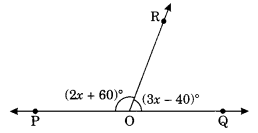LINES AND ANGLES
REVISION TEST
F.M=20
Q1: If two lines intersect at a point, then the vertically opposite angles are always
1 point
Q2: Two angles forming a linear pair are
1 point
Q3: A line that intersects two or more other lines at distinct points is called
1 point
Q4: If line l II m, then ∠1 = ∠ 2 because they are
1 point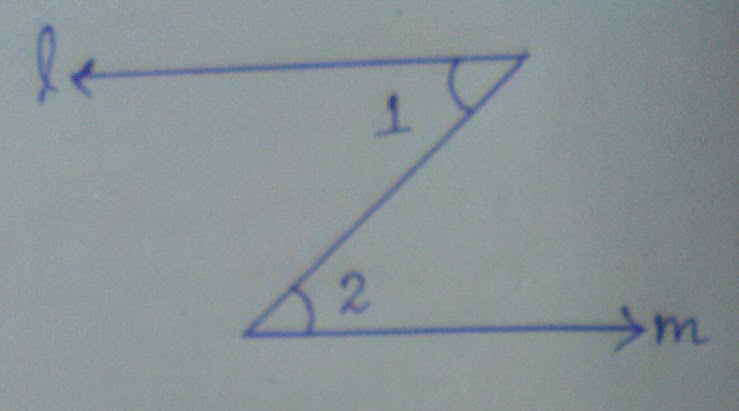Q5: If two parallel lines are cut by a transversal, each pair of the corresponding angles are _________ in measure.
1 point
Q6: Line a II b, c is a transversal then ∠y=?
1 point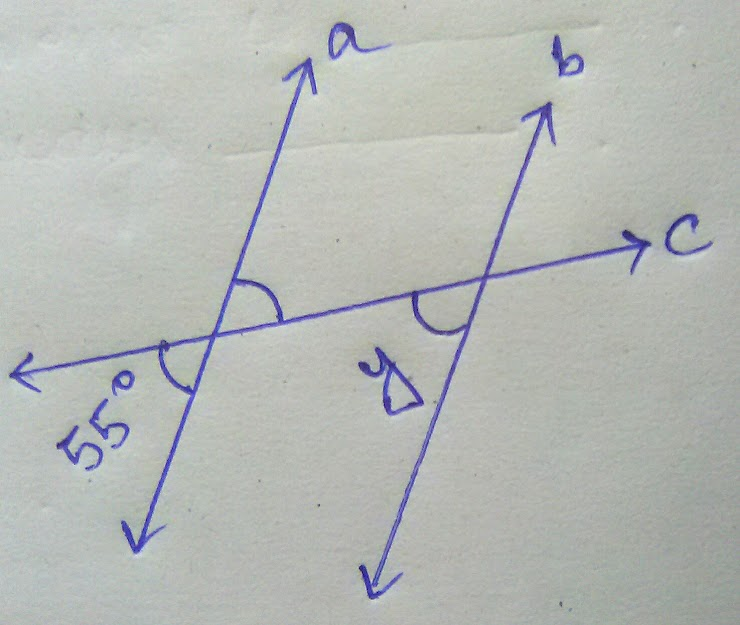Q7: The difference in the measures of two complementary angles is 12º. Find the measures of the angles.
2 points
Q8: Line l II m, t is a transversal, then ∠x=?
2 points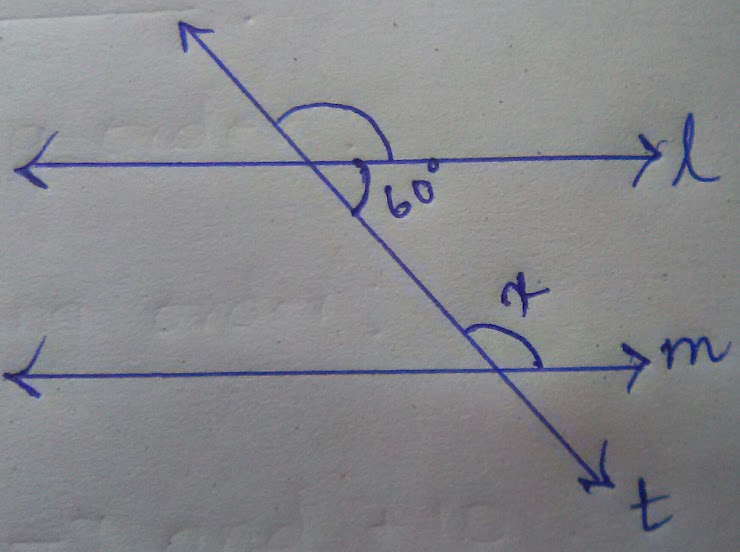Q9: Find the angle which is equal to its complement.
2 points
Q10: In given figure, AB II CD, find x and y.
2 points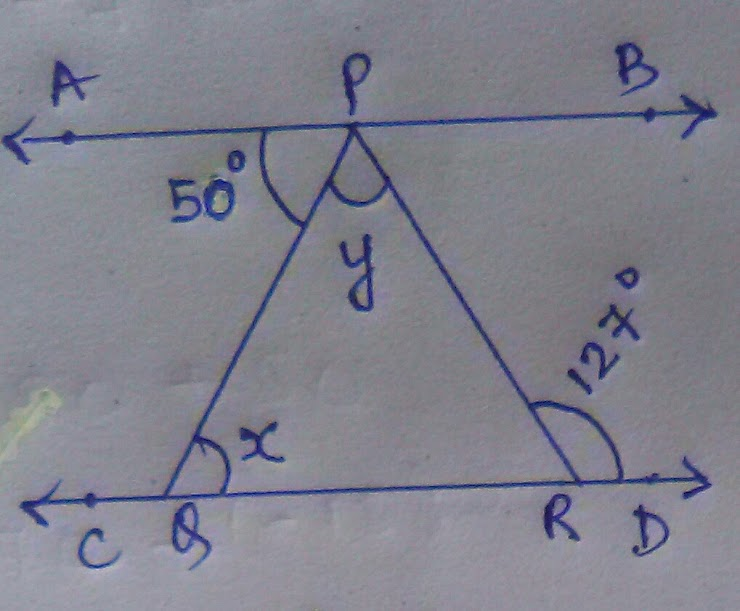Q11: The value of 'y' is
2 points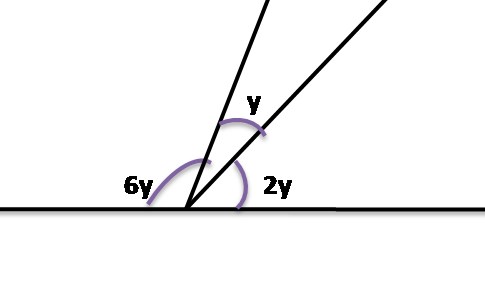Q12: The value of ∠x=?
2 points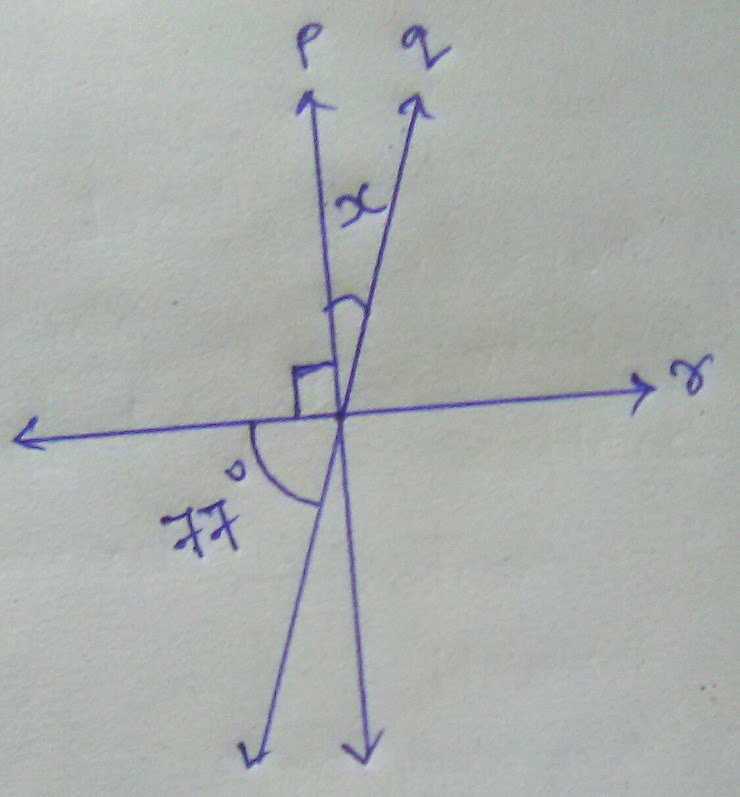Q13: The value of ∠POR is
2 points